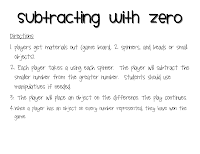Sunday, September 8, 2013

So much of first grade math is learning how to add and subtract.  In my class, we just finished our introductory unit of adding so now we are on to subtraction.

Here is a free activity to get you started too!

Here are some of my old freebies for subtraction!

This is a game to use with Candy Land.  The students pull a math card and if they can find the missing number in either an addition or subtraction problem then they get to select one of the game's card and move that amount of spaces on the board.  There will be more of these to come, so check back for them!

Spin, Subtract and Color, provided two spinners for each student. I have mine labeled 1 & 2.  On 1, I have numbers 11-18 and on 2, I have numbers labeled 0-10 (purchased at Dollar Tree).  The students spin and subtract and then color the difference.
Shinning Stars Subtraction- Each student needs a playing mat (several are included), cubes, a die and a recording sheet.  Directions are attached.